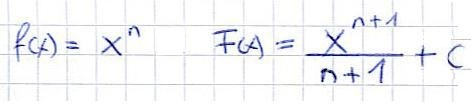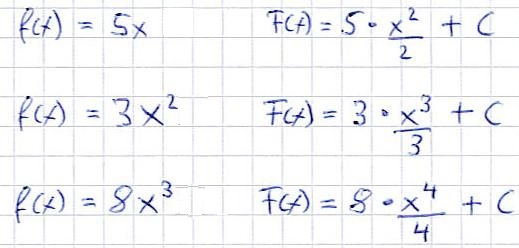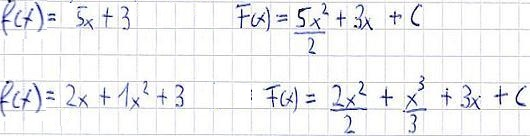# Why we use a constant in integration

## Integrate: antiderivative, power rule and sum rule

In this section we deal with finding antiderivatives and the basic rules of integration. We will first concentrate on the factor rule and the sum rule.

First of all, if you don't know what integration is all about, you should first work through our article Basics of Integration. This knowledge is needed to understand what an antiderivative is and how to find it.

Show:

### Indefinite integral

You certainly still know functions. For example, there was: f (x) = y = 2x or f (x) = y = 2x3 + 3x. And then there were the derivatives, for example f '(x) = y' = 2 or f '(x) = y' = 6x2 + 3. When integrating, we go in the opposite direction. We have a function and we integrate it. The result is an antiderivative. So again to take notes: We have a function y = f (x) and are looking for the antiderivative Y = F (x).

One more hint: We will now start with the integration rules without considering integration limits! The first thing to do here is to find the antiderivative. We then use the limits. We turn to the integration limits in the area calculation chapter.

Show:

### Integrate constant / power rule

Let's start with the integration with the power rule. First a constant is integrated here:

• f (x) = 2 dx -> F (x) = 2x + C
• f (x) = 5 dx -> F (x) = 5x + C
• f (x) = 8 dx -> F (x) = 8x + C

Note: A constant is integrated by adding an "x" to the constant and writing + C. The C stands for any number. Let this C stand as it is for now. The reason: If you derive 2x + 2 or 2x + 5 or generally 2x + C, you get f (x) = 2 again.

Power rule:

Now we want functions such as f (x) = 2x or f (x) = 3x2 integrate. To do this, we use the power rule, which looks like this:The application of the power rule is actually quite simple. Look at the exponent of the function you want to incorporate. Add the number 1 to this and you have the new exponent and the new number below the fraction. Some examples:Another small note: In general, a "dx" is written after the function, for example f (x) = (5x) dx. This means that the function is integrated with respect to x. In order to work mathematically correct now, we will also use this notation in the following examples.

### Sum rule

As with the sum rule of differentiation, there is also a sum rule with integration that looks very similar. This says that you are allowed to integrate the limbs. As always, some examples are probably best for understanding:Left:

### Who's Online

We have 190 guests online